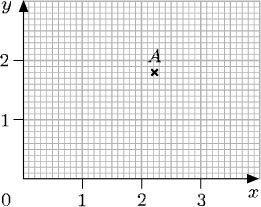A great advantage of the metric system of units is that conversion between units within the system is particularly easy. For example, ‘£1 is worth 100p’ is converting one pound into pence. To convert pounds to pence, you multiply by 100. So £2 is 200p, and £2.63 is 263p. (Remember that to multiply by 100, you move the digits two places to the left in the place value table.)

To convert from pence to pounds, you need to reverse this process, i.e. to divide by 100 (moving the
Author(s): The Open University

## Activity 15

Suggest appropriate units for each of the following:

• (a) the age of the kitten when it is weaned;

• (b) the distance between one train station and the
Author(s): The Open University

## Activity 14

Contour lines on a map show all the points at a given height above sea level. The lines are drawn for each height at 50-metre intervals, and points below sea level are shown by negative heights. The diagra
Author(s): The Open University

Numbers can be positive or negative, i.e. greater than or less than zero. Negative numbers have several uses; for example, to measure temperatures below zero, such as 3°C (‘minus 3 degrees Celsius’). They are also used to represent debts and overdrawn accounts: a bank balance of £84.33 means ‘overdrawn by £84.33’.

Negative numbers are shown on the number line to the left of 0. The animation below shows 8, 7,
Author(s): The Open University

A fraction is written as one number over another (such as) and means the top number divided by the bottom number. The top number, 3, is called the numerator and the bottom number, 10, is call
Author(s): The Open University

## Activity 6

What is 370.76 grams in kilograms? There are 1000 grams in a kilogram.

370.76 ÷ 1000 = 0.370 76.

So 370.76 g = 0.370
Author(s): The Open University

## Activity 2

Write each of the following three numbers in numerals and then place them in ascending order:

• eight hundred and eight thousand

• two million and twenty
Author(s): The Open University

## Activity 1

Can you answer the following questions:

• (a) Write ‘twenty thousand one hundred and forty-four’ as a number.

• (b) Say (or write) the number 31 002
Author(s): The Open University

After studying this course, you should be able to:

• write whole numbers and decimals in place-value columns and compare their sizes

• multiply and divide whole numbers and decimals by 10, 100, 1000 and so on

• indicate given fractions on a diagram and find equivalent fractions for a given fraction

• mark numbers on a number line

• choose appropriate units for a given purpose.

Author(s): The Open University

The content acknowledged below is Proprietary (see terms and conditions) and is made available under a Creative Commons Attribution-NonCommercial-ShareAlike 4.0 Licence

Grateful acknowledgement is made to the following sources for permission to reproduce material in this course:<
Author(s): The Open University## Study another free course

There are more than 800 courses on OpenLearn for you to
Author(s): The Open University

Now try the quiz and see if there are any areas you need to work on.

Author(s): The Open University

## Activity 22

Write down the coordinates of the point P on each of the graphs below and interpret these coordinates in terms of the labels on the axes.

Author(s): The Open University

## Activity 20

Look at the diagram below and answer the following questions:

• (a) Write down the coordinates of the points P, Q, R, S and T.

Author(s): The Open University

Where necessary, the coordinates of a point can be specified by using decimals or fractions. However, locating points whose coordinates are not whole numbers requires more precision when reading along the axes. For example, here the coordinates of the point A are (2.2, 1.8).Author(s): The Open University

Up to now only those points with positive or zero coordinates have been considered. But the system can be made to cope with points involving negative coordinates, such as (−2, 3) or (−2, −3). Just as a number line can be extended to deal with negative numbers, the x-axis and y-axis can be extended to deal with negative coordinates.

[Image_Link]https://www.open.edu/openlearn/ocw/pluginfile.php/94235/mod_oucont
Author(s): The Open University

## Activity 16

Write down the coordinates of A and B.

[Image_Link]https://www.open.edu/openlearn/ocw/pluginfile.php/94235/mod_oucontent/oucont
Author(s): The Open University

For many towns and cities, an individual book of street maps called an A to Z has been produced. You can look up the name of a street in the index, and it will give you the page number of the map that contains the street, plus the grid reference square for the street. There are different conventions for these grid references. You may have met several of these.